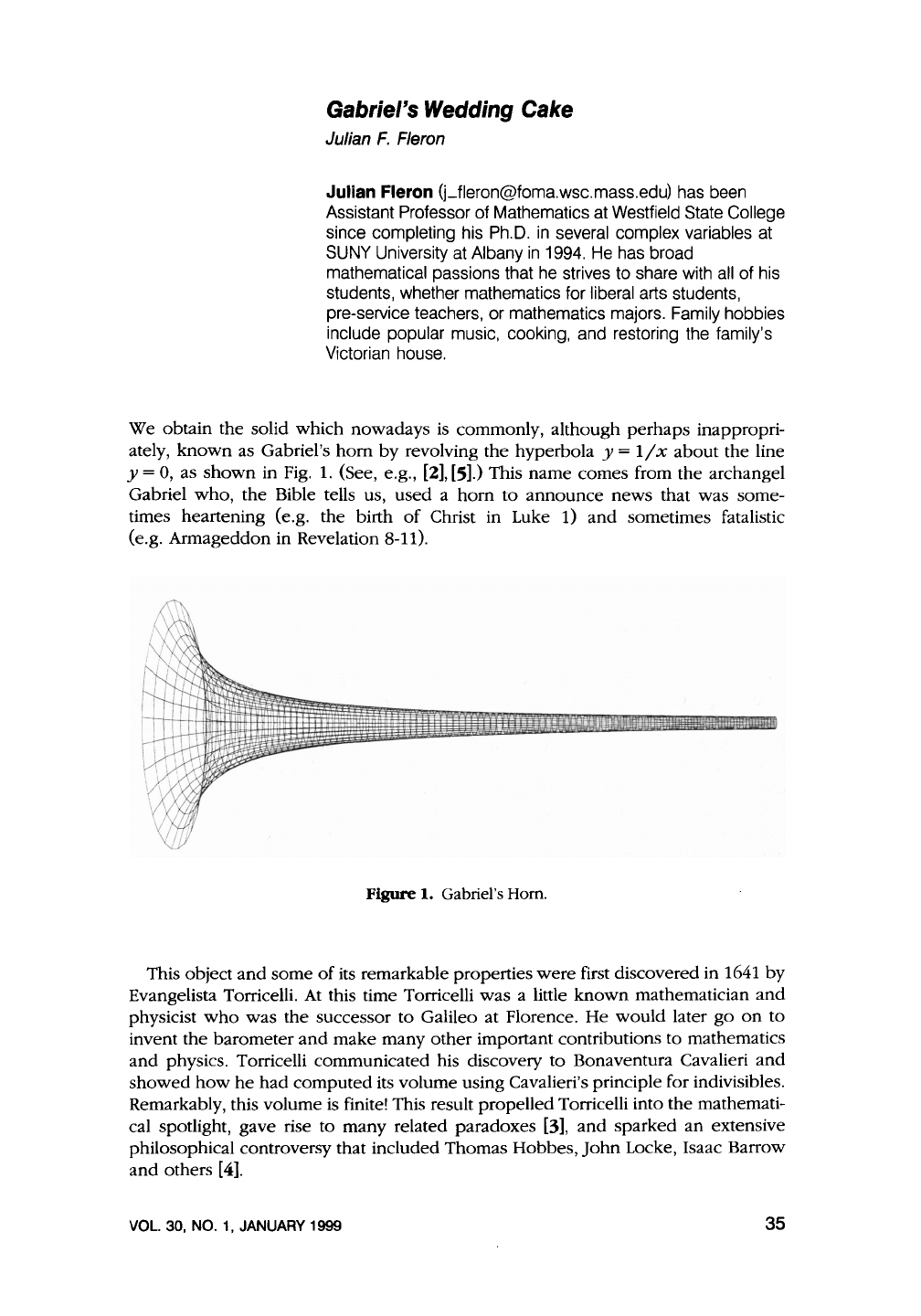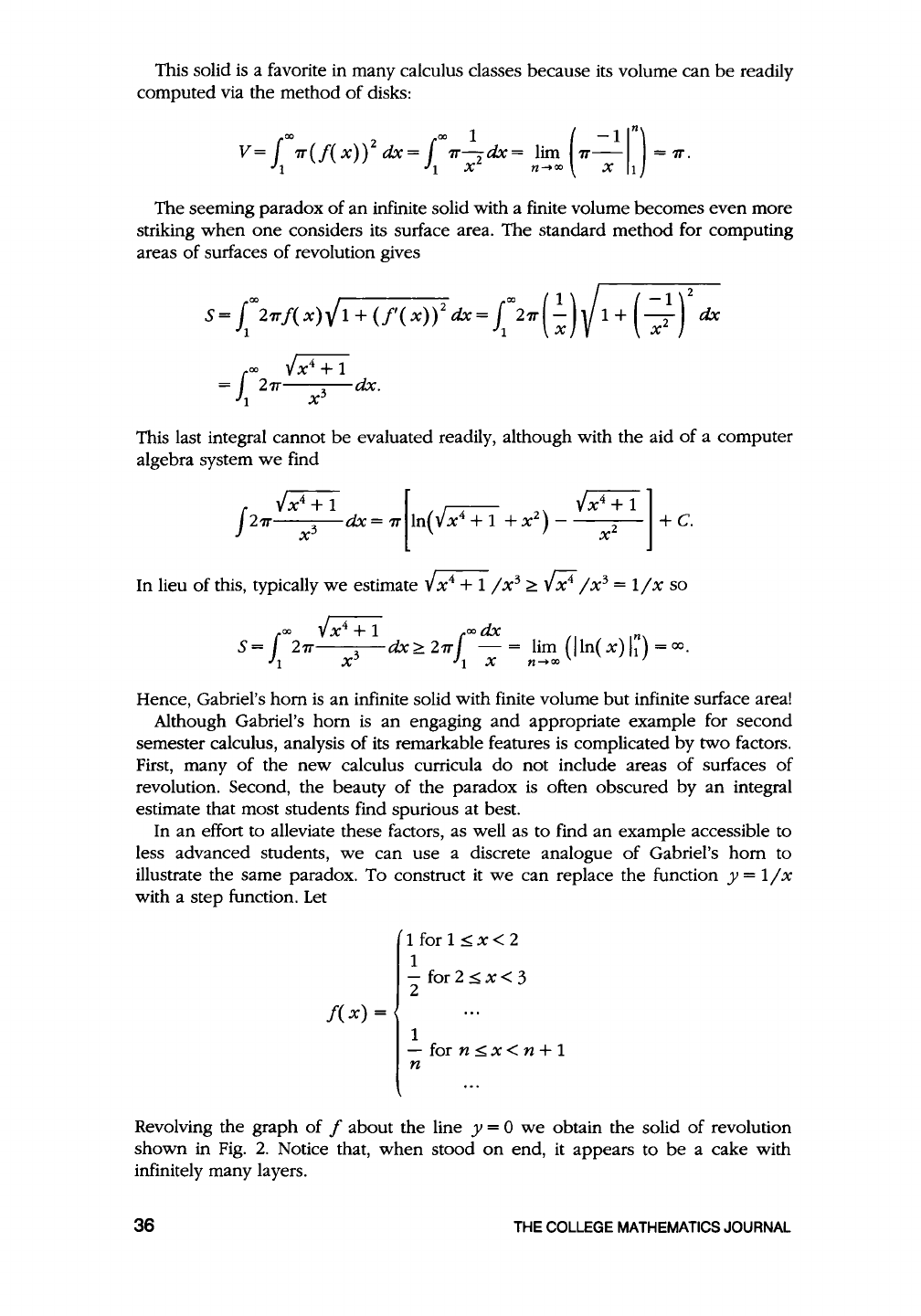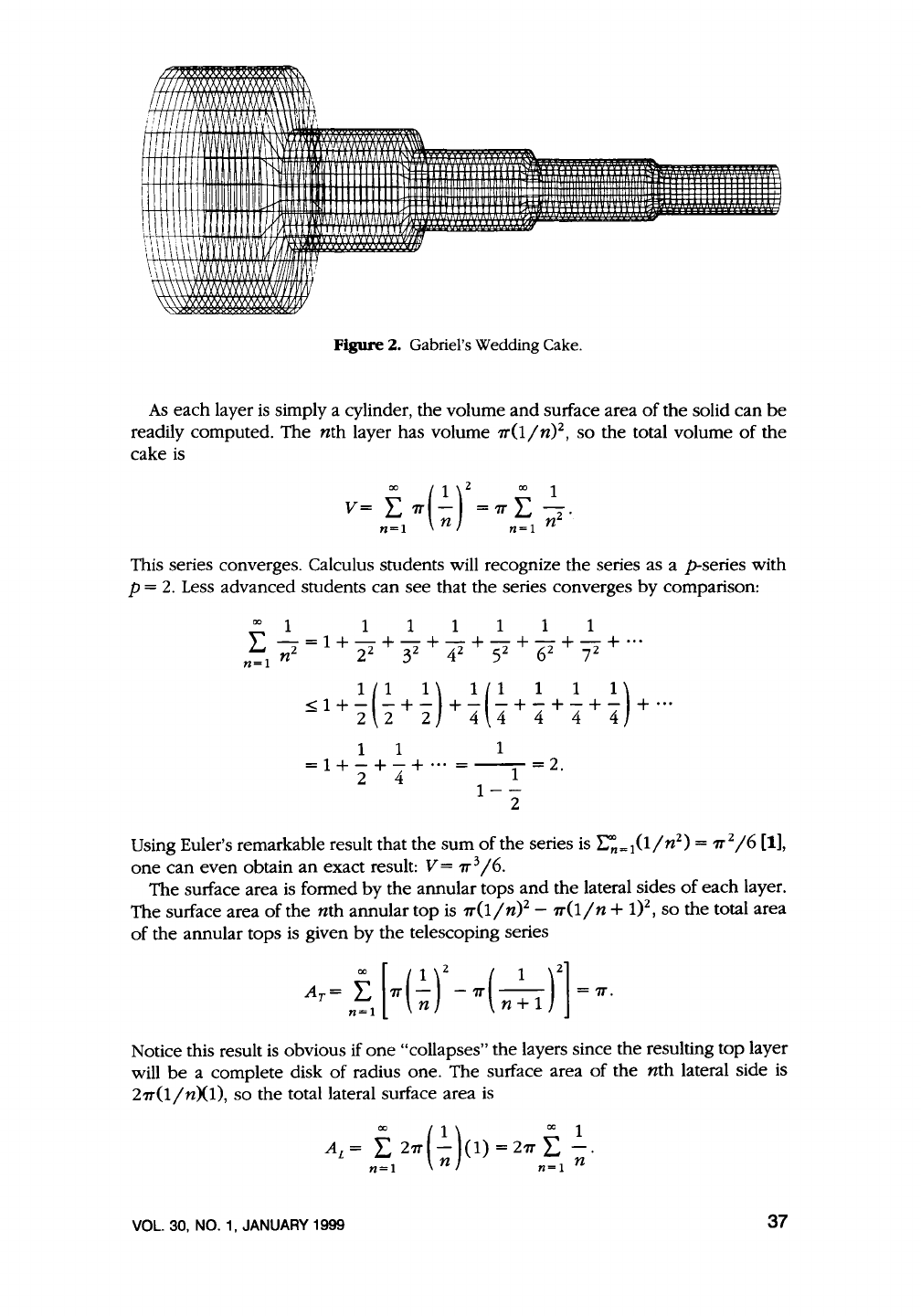#### TL;DR Gabriel's wedding cake describes a geometric figure w...
Three-dimensional illustration of Gabriel’s Horn. This horn shape h...
**Cavalieri's Principle:** states that if two objects are between t...
Determining the surface of the horn is a slightly more complex than...
How to transform the the "continuous horn" into a "discrete cake".Gabriel's
Wedding
Cake
Julian
F.
Fleron
Julian Fleron (j_fleron@foma.wsc.mass.edu) has been
Assistant Professor of Mathematics at
Westfield State College
since completing his Ph.D.
in
several complex variables at
SUNY University at
Albany
in
mathematical passions that he strives to share with all of his
students, whether mathematics for
liberal arts students,
pre-service teachers, or mathematics majors.
Family hobbies
include popular music, cooking, and restoring the family's
Victorian house.
We obtain the solid which nowadays
is
commonly, although
perhaps
inappropri-
ately,
known
as Gabriel's
hom
by
revolving
the
hyperbola y =
1/x
the line
y = 0, as
shown
in
Fig.
1.
(See, e.g.,
,
.) This
name
comes from the archangel
Gabriel
who,
the
Bible tells us,
used
a
hom
to
announce
news
that was some-
times heartening (e.g. the birth
of
Christ in Luke 1)
and
sometimes fatalistic
(e.g. Armageddon in Revelation
8-11).
Figure 1. Gabriel's
Horn.
This object
and
some
of
its remarkable properties
were
first discovered in
1641
by
Evangelista Torricelli.
At
this time Torricelli was a little
known
mathematician
and
physicist
who
was the successor to Galileo at Florence.
He
would
later
go
on
to
invent
the
barometer
and
make
many
other
important contributions to mathematics
and
physics. Torricelli communicated his discovery
to
Bonaventura Cavalieri
and
showed
how
he
computed
its volume using Cavalieri's principle for indivisibles.
Remarkably, this volume
is
finite! This result propelled Torricelli into the mathemati-
cal spotlight, gave rise to
many
,
and
sparked
an
extensive
philosophical controversy that included Thomas Hobbes,
John
Locke, Isaac Barrow
and
others [
4].
VOL. 30, NO. 1, JANUARY 1999
35This solid
is
a favorite in many calculus classes because its volume can
be
computed via the method
of
disks:
co co
1 (
-1
In)
V=
1 7T(f(
x)
)
2
dx
= 1
7T-
2
dx
= lim
7r-
=
7T.
1 1 X X 1
of
an
infinite solid with a finite volume becomes even more
striking
when
one
considers its surface area. The standard method for computing
areas
of
surfaces
of
revolution gives
This last integral cannot
be
evaluated readily, although with the aid
of
a computer
algebra system
we
find
In lieu
of
this, typically
we
estimate
...J
x
4
+ 1 I x
3
0 I x
3
= 1 I x so
co
...J
x
4
+ 1
co
dx
n
5=1
27T
3
-=lim
(lln{x)lt)=oo.
1 X 1 X
Hence, Gabriel's
hom
is
an
infinite solid with finite volume but infinite surface area!
Although Gabriel's
hom
is
an
engaging
and
appropriate example for second
semester calculus, analysis
of
its remarkable features
is
complicated by two factors.
First, many
of
the
new
calculus curricula
do
not include areas
of
surfaces
of
revolution. Second, the beauty
of
is
often obscured
by
an
integral
estimate that most students find spurious at best.
In
an
effort to alleviate these factors, as well as to find
an
example accessible to
we
can use a discrete analogue
of
Gabriel's
hom
to
illustrate the same paradox. To construct
it
we
can replace the function y =
11x
with a step function.
Let
f(x)
=
1 for 1 2
1
-
2
1
- for n x < n + 1
n
Revolving the graph
of
f about the line y = 0
we
obtain the solid
of
revolution
shown in
Fig.
2.
Notice that,
when
stood
on
end, it appears to
be
a cake with
infinitely many layers.
36
THE
COLLEGE
MATHEMATICS
JOURNAL/////
(1/////'A.
\XXXXXX\\'
N\
:
1
//////!'
IV
,v,,
,:"\n '
•'\
II 1
\\\\\\
'11
l
U/111!
cxl/f
11
Figure
2. Gabriel's Wedding Cake.
As
each layer
is
simply a cylinder, the volume and surface area
of
the solid can
be
nth
layer has volume
1T(lln)
2
,
so
the total volume
of
the
cake
is
V=
i:
1T(2_)
2
=
1T
E
\.
n=I
n
n=I
n
This series converges. Calculus students will recognize the series as a p-series with
p =
2.
Less advanced students can see that the series converges
by
comparison:
00
1
111111
"-=1+-+-+-+-+-+-+
...
n'":I
nz
22
32
42
52
62
72
< 1 +
2.(2.
+
2.)
+
2.(2.
+..:. +..:. +
2.)
+ ...
-22
2
44
4 4 4
1 1 1
=
1
+ 2 + 4 + ... =
--1-
=
2
·
1--
2
Using Euler's remarkable result that the
sum
of
the series
is
E:=
1
(1ln
2
)
=
1r
2
16
,
one
can even obtain
an
exact result:
V=
1r
3
16.
The surface area
is
formed
by
the annular tops
and
the lateral sides
of
each layer.
The surface area
of
the
nth
annular top
is
7T(1
I n)
2
-
1T(l
In
+ 1)
2
,
so
the total area
of
the annular tops
is
given by the telescoping series
Notice this result
is
obvious if
one
"collapses" the layers since the resulting top layer
will
be
a complete disk
of